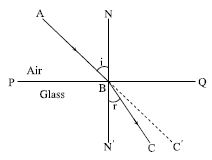Physics

# Describe Laws of Refraction of Light

We have already noticed that in the figure AB is the incident ray, BC is the refracted ray and NBN’ is the normal drawn at B on PQ. ˄ABN is the angle of incidence i and ˄N’BC is the angle of refraction r.Now if the angle of incidence is increased the angle of refraction will also increase but the angle of refraction will not be proportional to the angle of incidence that is if the angle of incidence i is doubled the angle of refraction r will never be so. It is seen that if the angles of incidence are i1, i2, i3……… and their respective angles of refraction are:

r1, r2, r3, ……….. then [sin i1/sin r1] = [sin i2/sin r2] = [sin i3/sin r3] = constant.

First law: The incident ray, normal drawn at the point of incidence on the surface of separation and the refracted ray lie on the same plane.

Second law: When light enters obliquely to a transparent medium from another transparent medium then for a fixed pair of media and for a fixed colour of light the ratio of the sine of the angle of incidence to the sine of the angle of refraction always remains constant.

This law is also known as Snell’s law.